## 作业要求一 （20分）

1）C高级第三次PTA作业（1）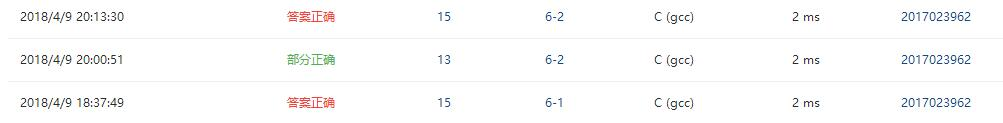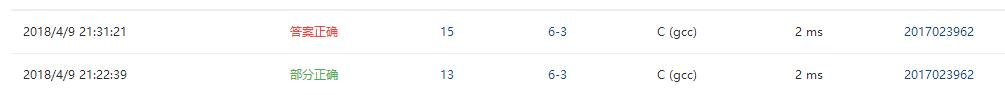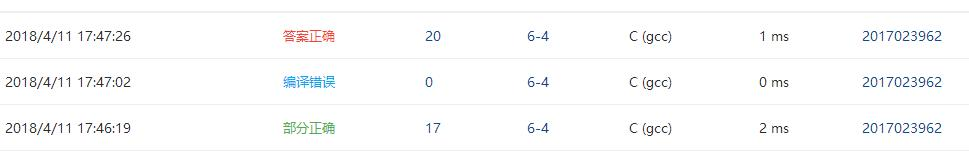2）C高级第三次PTA作业（2）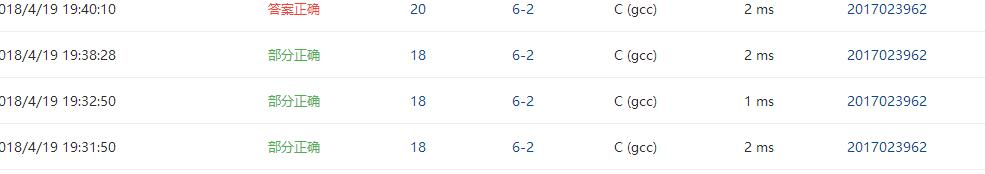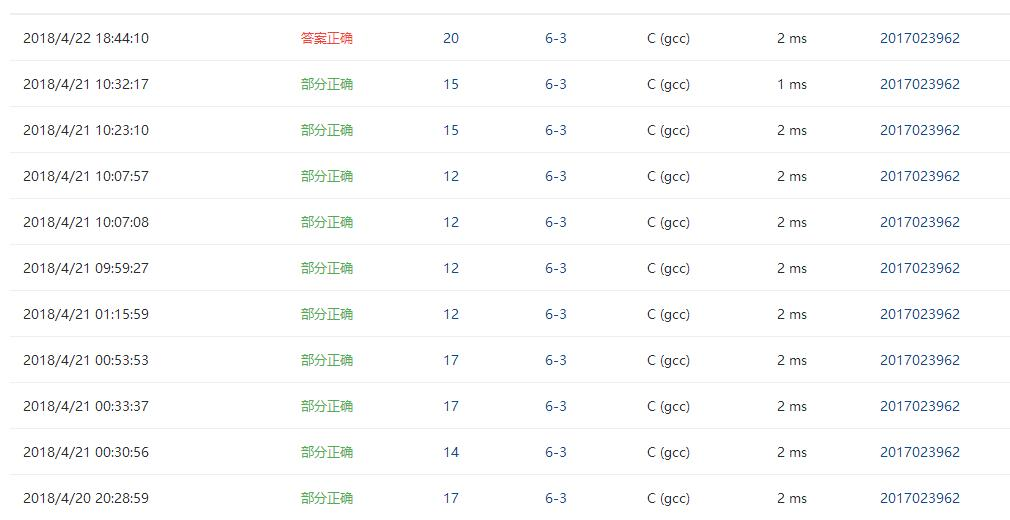3）一道编程题：

## 作业要求二（65分）

1.设计思路（6分）
(1)主要描述题目算法（2分）。

(2)流程图（4分）
2.实验代码（2分）

char *getmonth( int n )
{

if(n>12||n<1)
{return 0;
}
else
{
switch (n)
{case 1:return "January" ;
case 2:return "February"  ;
case 3:return "March";
case 4:return "April" ;
case 5:return "May" ;
case 6:return "June" ;
case 7:return "July";
case 8:return "August";
case 9:return "September";
case 10:return "October";
case 11:return "November";
case 12:return "December" ;
}
}
}


3.本题调试过程碰到问题及解决办法（4分）

1.设计思路（6分）
(1)主要描述题目算法（2分）。

(2)流程图（4分）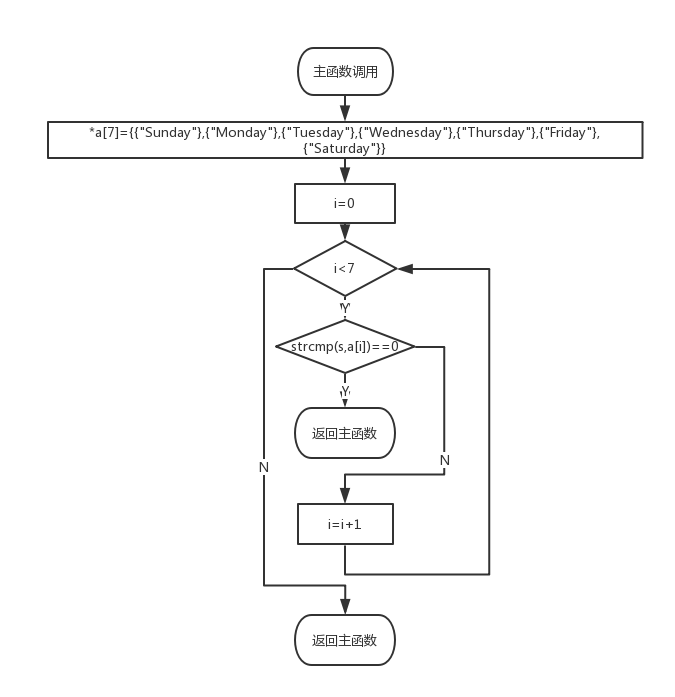2.实验代码（2分）

int getindex( char *s )
{   int i;
char *a={{"Sunday"},{"Monday"},{"Tuesday"},{"Wednesday"},{"Thursday"},{"Friday"},{"Saturday"}};
for(i=0;i<7;i++)
{if(strcmp(s,a[i])==0)
{
return i;
}
}
return -1;

}


3.本题调试过程碰到问题及解决办法（4分）

1.设计思路（6分）
(1)主要描述题目算法（2分）。

(2)流程图（4分）
2.实验代码（2分）

int max_len( char *s[], int n )
{   int i;
int t;
int length;
int max=strlen(s);
for(i=0;i<n;i++)
{ length=strlen(s[i]);
if(max<length)
{
t=length;
length=max;
max=t;
}
}

return max;
}


3.本题调试过程碰到问题及解决办法（4分）

1.设计思路（6分）
(1)主要描述题目算法（2分）。

(2)流程图（4分）
2.实验代码（2分）

char *match( char *s, char ch1, char ch2 )
{   int i,m=0;
char *q;
int mark=-1,flag=-1;
int biaoji1=0,biaoji2=0;
for(i=0;;i++)
{if(*(s+i)=='\0')
{break;
}
if(*(s+i)==ch1&&mark==-1)
{mark=i;
}
if(*(s+i)==ch2)
{if(biaoji2!=1||i<biaoji1)
{ flag=i;
biaoji2=1;
biaoji1=flag;
}
}
}
if(mark==-1)
{
printf("\n");
*s='\0';
return s;
}
if(mark!=-1&&flag!=-1)
{
for(i=mark;i<=flag;i++)
{
if(i==flag)
{printf("%c\n",*(s+i));
}
else
{   printf("%c",*(s+i));
}
}
return (s+mark);
}
if(mark!=-1&&flag==-1)
{
printf("%s\n",(s+mark));
return (s+mark);
}
}


3.本题调试过程碰到问题及解决办法（4分）

6-1 奇数值结点链表

1.设计思路（6分）
(1)主要描述题目算法（2分）。

(2)流程图（4分）
2.实验代码（2分）

struct ListNode *readlist()
{  int i;

for(i=0;;i++)
{t=(struct ListNode*)malloc(sizeof(struct ListNode));
scanf("%d",&t->data);
if(t->data==-1)
{end->next=NULL;

break;
}
{
end=t;
}
else
{end->next=t;
end=t;
}

}
}
struct ListNode *getodd( struct ListNode **L )
{  struct ListNode *start1,*a,*b,*c,*start2;
a=*L;
start1=(struct ListNode*)malloc(sizeof(struct ListNode));
start2=(struct ListNode*)malloc(sizeof(struct ListNode));
start1->next=NULL;
start2->next=NULL;
b=start1;
c=start2;
for(;;)
{
if(a==NULL)
{
break;
}
if(a->data%2!=0)
{
b->next=a;
b=a;
}
else
{
c->next=a;
c=a;
}
a=a->next;

}
c->next=NULL;
b->next=NULL;
*L=start2->next;
return start1->next;
}


3.本题调试过程碰到问题及解决办法（4分）

6-2 学生成绩链表处理

1.设计思路（6分）
(1)主要描述题目算法（2分）。

(2)流程图（4分）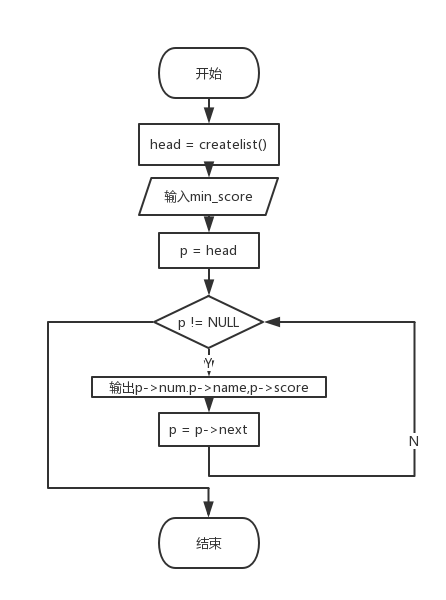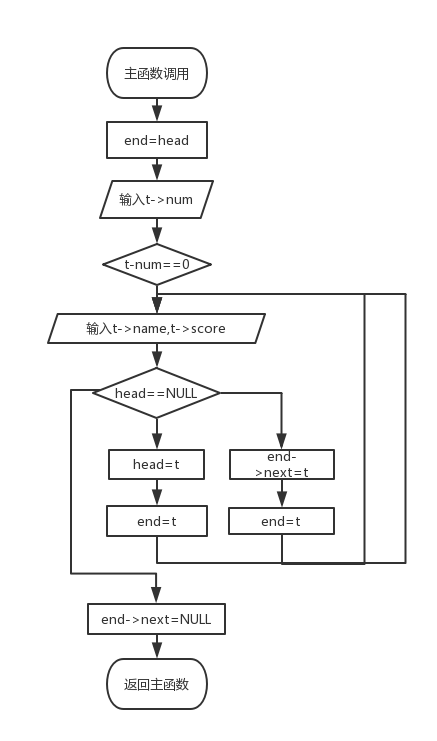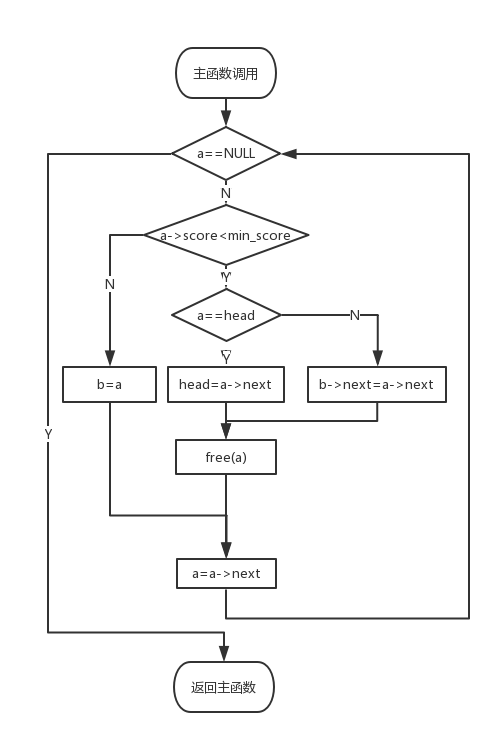2.实验代码（2分）

struct stud_node *createlist()
{
for(;;)
{ t=(struct stud_node*)malloc(sizeof(struct stud_node));
scanf("%d",&t->num);
if(t->num==0)
{
break;
}
scanf("%s",t->name);
scanf("%d",&t->score);

end=t;
}
else
{end->next=t;
end=t;
}
}
end->next=NULL;
}
struct stud_node *deletelist( struct stud_node *head, int min_score )
{
for(;;a=a->next)
{   if(a==NULL)
{break;
}
if(a->score<min_score)
free(a);
}
else
{
b->next=a->next;
free(a);
}
}
else
{b=a;
}

}
}


3.本题调试过程碰到问题及解决办法（4分）

6-3 链表拼接

1.设计思路（6分）
(1)主要描述题目算法（2分）。

(2)流程图（4分）
2.实验代码（2分）

struct ListNode *mergelists(struct ListNode *list1, struct ListNode *list2)
{
int num = 0;
int temp;
struct ListNode  *p = list1;
while(p != NULL)
{
temp[num] = p->data;
num++;
p = p->next;
}
p = list2;
while(p != NULL)
{
temp[num] = p->data;
num++;
p = p->next;
}
int i,j;
for(i = 0; i < num; i++)
for(j = i + 1; j < num; j++)
{
if(temp[i] > temp[j])
{
int t;
t = temp[i];
temp[i] = temp[j];
temp[j] = t;
}
}
struct ListNode  *newlist = NULL;
struct ListNode  *endlist = NULL;
struct ListNode  *q;
for(i = 0; i < num; i++)
{
q = (struct ListNode  *)malloc(sizeof(struct ListNode));
q->data = temp[i];
if(newlist == NULL)
{
newlist = q;
newlist->next = NULL;
}
if(endlist != NULL)
{
endlist->next = q;
}
endlist = q;
endlist->next = NULL;
}
return newlist;
}



3.本题调试过程碰到问题及解决办法（4分）

## 要求三、学习总结和进度（15分）

1、总结两周里所学的知识点，回答下列问题？（用自己的话表达出你的理解，网上复制粘贴没有分数）（5分）


char *getmonth( int n )
{
char *month={"January","Febuary","March","April","May","June","July","August","September","October","November","December"};
char **p;
p= month;
int i=0;
for(i=1;i<=12;i++)
{
if(n==i)
{
return *(p+i-1);
}
}
if(n>=13||n<=0)
{
return NULL;
}
}



2、将PTA作业的源代码使用git提交到托管平台上，要求给出上传成功截图和你的git地址。

3、点评3个同学的本周作业

4、请用表格和折线图呈现你本周（4/9 8:00~4/23 8:00）的代码行数和所用时间、博客字数和所用时间（3分）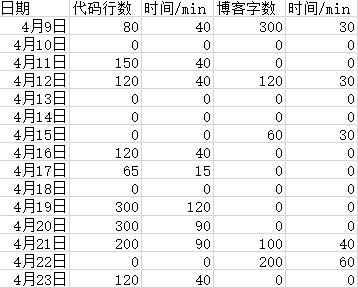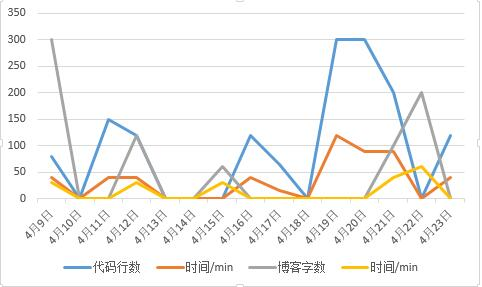posted on 2018-04-22 19:50  徐铭博  阅读(294)  评论(3编辑  收藏  举报